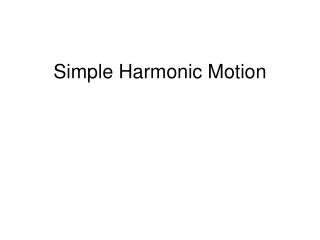DownloadDownload PresentationSimple Harmonic Motion

# Simple Harmonic Motion

Download Presentation## Simple Harmonic Motion

- - - - - - - - - - - - - - - - - - - - - - - - - - - E N D - - - - - - - - - - - - - - - - - - - - - - - - - - -
##### Presentation Transcript

1. Simple Harmonic Motion

2. Spring Constant, K The constant k is called the spring constant. SI unit of k = N/m.

3. HOOKE'S LAW The restoring force of an ideal spring is given by, where k is the spring constant and x is the displacement of the spring from its unstrained length. The minus sign indicates that the restoring force always points in a direction opposite to the displacement of the spring.

4. Simple Harmonic Motion When there is a restoring force, F = -kx, simple harmonic motion occurs.

5. Position VS. Time graph

6. Amplitude Amplitude is the magnitude of the maximum displacement.

7. Period, T For any object in simple harmonic motion, the time required to complete one cycle is the period T.

8. Frequency, f The frequency f of the simple harmonic motion is the number of cycles of the motion per second.

9. Oscillating Mass Consider a mass m attached to the end of a spring as shown. If the mass is pulled down and released, it will undergo simple harmonic motion. The period depends on the spring constant, k and the mass m, as given below,

10. How Do Astronauts Weigh Themselves While in Orbit? Astronauts who spend long periods of time in orbit periodically measure their body masses as part of their health-maintenance programs.

11. How Do Astronauts Weigh Themselves While in Orbit? Astronauts who spend long periods of time in orbit periodically measure their body masses as part of their health-maintenance programs. On earth, it is simple to measure body mass, with a scale. However, this procedure does not work in orbit, because both the scale and the astronaut are in free-fall and cannot press against each other.

12. Mass of an Astronaut This device consists of a spring-mounted chair in which the astronaut sits. The chair is then started oscillating in simple harmonic motion. The period of the motion is measured electronically and is automatically converted into a value of the astronaut’s mass, after the mass of the chair is taken into account.